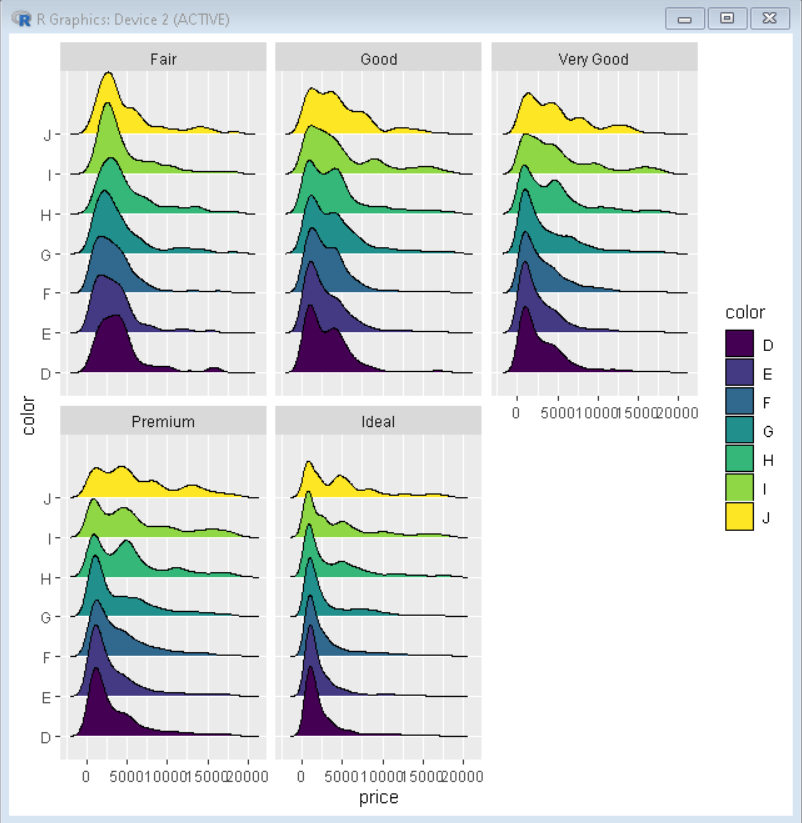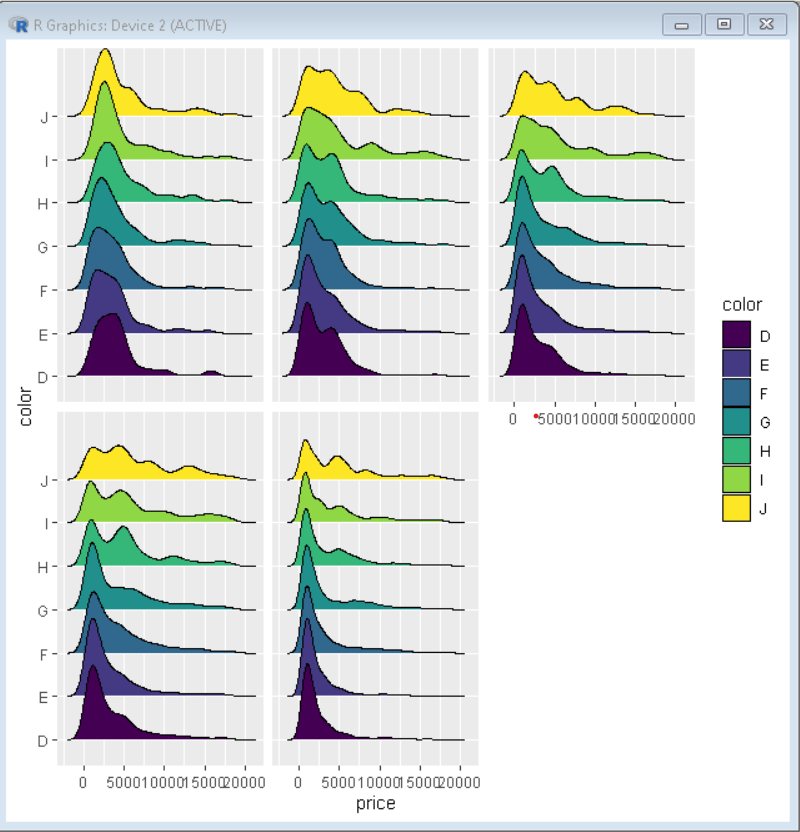# Remove Labels from ggplot2 Facet Plot in R

• Last Updated : 24 Oct, 2021

In this article, we will discuss how to remove the labels from the facet plot in ggplot2 in the R Programming language.

Facet plots, where one subsets the data based on a categorical variable and makes a series of similar plots with the same scale. We can easily plot a facetted plot using the facet_wrap() function of the ggplot2 package. When we use facet_wrap() in ggplot2, by default it gives a title to each plot according to the group they are divided into.

Syntax:

`plot + facet_wrap( ~facet-variable)`

Parameter:

• facet-variable: Determines the variable around which plots have to be divided.

Here, is a basic facet plot made using the diamonds data frame which is provided by R Language natively. We have used the facet_wrap() function with ~clarity to divide the plot into facets according to their clarity.

Example: Basic plot

## R

 `# load library ggridges and tidyverse``library``(ggridges)``library``(tidyverse)`` ` `# Basic facet plot divided according to category cut``# ggplot() function is used to plot the chart``ggplot``(diamonds, ``aes``(x=price, y=color, fill=color)) +`` ` `# geom_density_ridges() function is used to draw ``# ridgeline plot``  ``geom_density_ridges``()+`` ` `# facet_wrap() function divides the plot in facets ``# according to category of cut``  ``facet_wrap``(~cut)`

Output:## Remove labels from Facet plot

We can customize various aspects of a ggplot2 using the theme() function. To remove the label from facet plot, we need to use “strip.text.x” argument inside the theme() layer with argument ‘element_blank()’.

Syntax:

`plot + theme( strip.text.x = element_blank() )`

Example: Removing the label from facet plot

## R

 `# load library ggridges and tidyverse``library``(ggridges)``library``(tidyverse)`` ` `# Basic facet plot divided according to category cut``# ggplot() function is used to plot the chart``ggplot``(diamonds, ``aes``(x=price, y=color, fill=color)) +`` ` `# geom_density_ridges() function is used to draw ``# ridgeline plot``  ``geom_density_ridges``()+`` ` `# facet_wrap() function divides the plot ``# in facets according to category of cut``  ``facet_wrap``(~cut)+``# strip.text.x parameter of theme``# function is used to remove the label of facet plot``# element_blank() removes the label``theme``(strip.text.x = ``element_blank``())`

Output:My Personal Notes arrow_drop_up